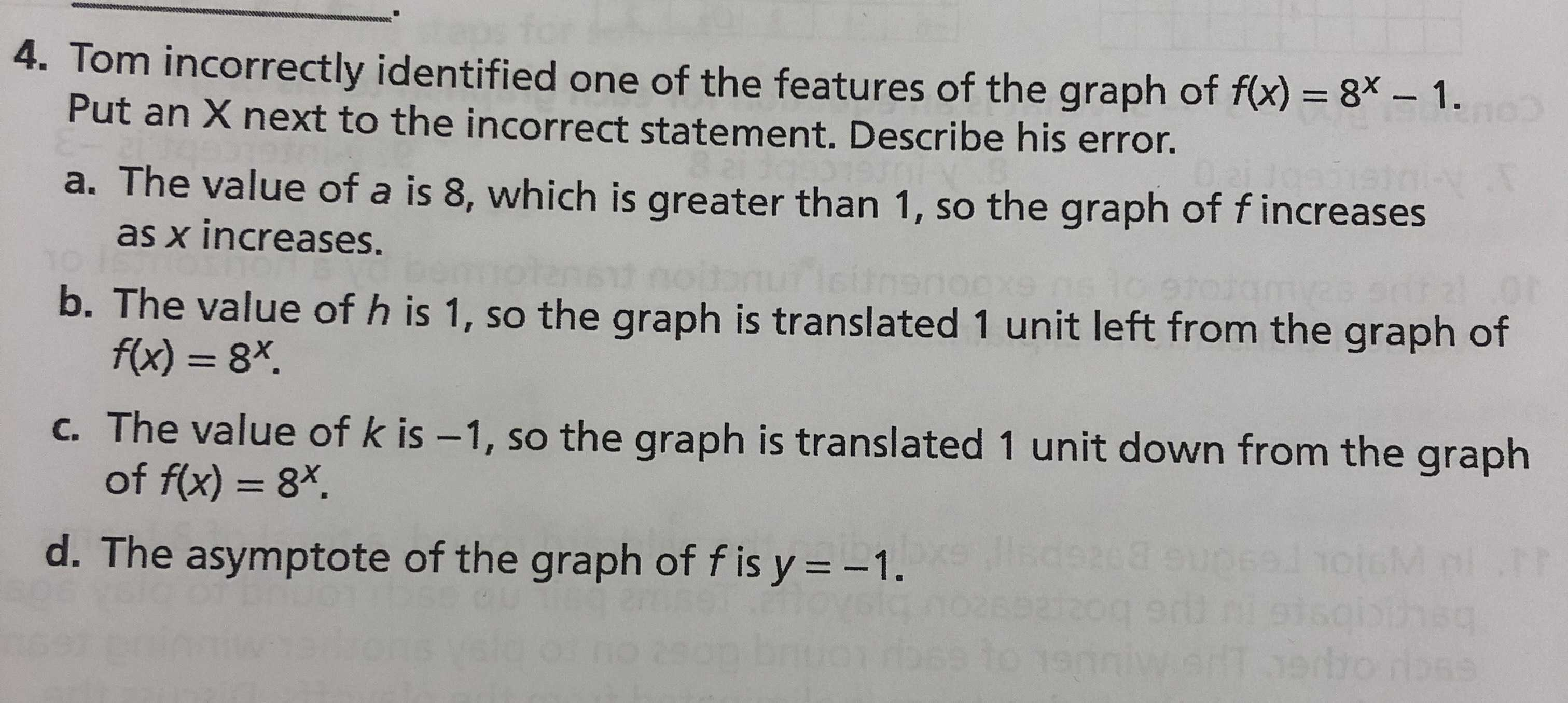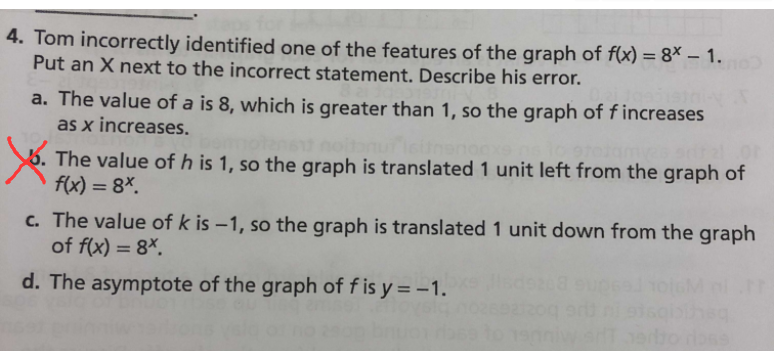### ¿Todavía tienes preguntas de matemáticas?

Pregunte a nuestros tutores expertos
Algebra
PreguntaTom incorrectly identified one of the features of the graph of $$f ( x ) = 8 ^ { x } - 1 .$$

Put an $$X$$ next to the incorrect statement. Describe his error. a. The value of a is $$8 ,$$ which is greater than $$1 ,$$ so the graph of $$f$$ increases as $$x$$ increases. b. The value of $$h$$ is $$1 ,$$ so the graph is translated $$1$$ unit left from the graph of

$$f ( x ) = 8 ^ { x }$$ . c. The value of $$k$$ is $$- 1 ,$$ so the graph is translated $$1$$ unit down from the graph of $$f ( x ) = 8 ^ { x }$$ . d. The asymptote of the graph of $$f$$ is $$y = - 1$$ .